# The other wayElectronics guide > Capacitors > The other way

During these experiments you should have noticed how the voltage across the capacitor appeared to stay for a while, even after the switch had been turned off. How could this be so?

Figure 4.11 shows the circuit of an experiment you should now do, which will help you to understand this unusual capability of capacitors to apparently store voltage. Build the circuit up as in the breadboard layout of Figure 4.12, with the switch in its on position.Figure 4.11 An experimental circuit to demonstrate how a capacitor stores voltage (or charge)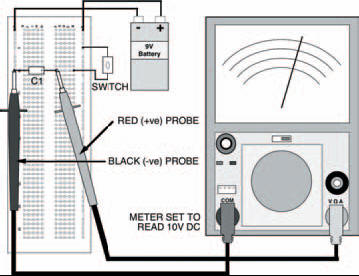Figure 4.12 The breadboard layout for the experiment shown in Figure 4.11

Now, measure the voltages at selected time intervals, and fill in the results into Table 4.5, then transfer them to form a curve in Figure 4.13, after switching the switch off.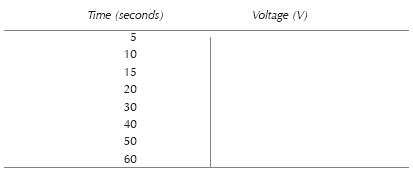Table 4.5 The results of your measurements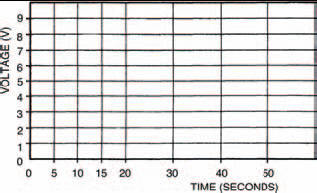Figure 4.13 A blank graph to plot your measurements. Use Table 4.5 shown above

The results we obtained in preparing this book are tabulated in Table 4.6 and plotted in Figure 4.14. You can see that the curve obtained is similar to the curves we got earlier, but are falling not rising. Like the earlier curves, this is an exponential curve, too.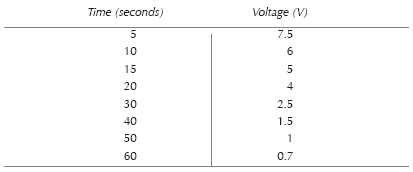Table 4.6 The results of our experiments while preparing this bookFigure 4.14 The graph showing our own results

If you wish, you can calculate the time constant here, in the same way, but remember that it must now be the time at which the voltage falls to 0.63 of the total voltage drop, that is, when: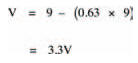<< Getting results